## 横向交流电场下液膜参数不稳定性分析1)

*北京航空航天大学宇航学院, 北京 100191

## PARAMETRIC INSTABILITY OF LIQUID SHEETS SUBJECTED TO A TRANSVERSE AC ELECTRIC FIELD1)

Wang Tiehan*, Fu Qingfei,*,,2), Yang Lijun*,

*School of Astronautics, Beihang University, Beijing 100191, China

Beijing Advanced Innovation Center for Big Date-based Precision Medicine, Beihang University, Beijing 100191, China

 基金资助: 1) 国家自然科学基金资助项目.  11922201

Received: 2020-08-25   Accepted: 2020-11-2   Online: 2021-02-07Abstract

Parametric resonance occurs at the gas-liquid interface when a liquid sheet moving in a transverse AC electrical field. In order to obtain the dispersion relation of liquid sheet under AC electric field and to provide the theoretical basis for the analysis of the breaking behavior of liquid sheet, in this paper, the temporal parametric instability under DC and AC electric field were both analyzed in the leaky dielectric model. The leaky dielectric model is used to characterize the electrical properties of liquid. Since the mean flow is time-dependent function, the Floquet theory is used to solve the stability problem. In this paper, the electric field is defined as a mixed electric field coupled by part of an ac electric field and part of a DC electric field. The dimensionless dispersion relation between wave number and temporal growth rate can be derived as a matrix. According to this relationship, the influence of various liquid properties on the parametric instability were discussed. The effects of the ratio of gas-to-liquid density ($\rho )$, the Weber number (We), the Reynolds number (Re), the electrical Euler number ($Eu$), the relative relaxation time ($\tau )$ and the characteristic of the proportion of AC electric field ($Pr$) and the frequency of the electric field ($\varOmega )$ was concluded in this paper. As a conclusion, the electrical Euler number ($Eu$) influence the instability of both capillary unstable and parametric unstable region, the proportion of electric field ($Pr$) effects as a constant electric field force, the frequency of the AC electric field ($\varOmega )$ mainly influence the parametric instability region. In the experiment, in order to obtain parameter oscillation phenomenon more easily, increasing electrical Euler number ($Eu$) and reducing the frequency of AC electric field ($\varOmega )$ are founded as effective methods.

Keywords： parametric instability ; liquid sheet ; electric field ; leaky dielectric model

Wang Tiehan, Fu Qingfei, Yang Lijun. PARAMETRIC INSTABILITY OF LIQUID SHEETS SUBJECTED TO A TRANSVERSE AC ELECTRIC FIELD1). Chinese Journal of Theoretical and Applied Mechanics[J], 2021, 53(2): 352-361 DOI:10.6052/0459-1879-20-300

## 1 理论推导

### 图1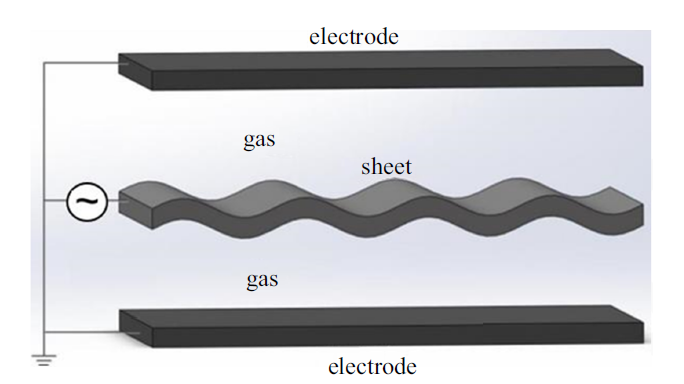Fig.1   Schematic diagram of moving liquid sheet in an AC electric field

### 1.2 直流电场作用下不稳定性分析

1.2.1 液相

$\frac{\partial u}{\partial x}+\frac{\partial v}{\partial y}=0,\ \ -a+\eta <y<a+\eta$
$\begin{array}{r}\rho\left[\frac{\partial u}{\partial t}+(U+u) \frac{\partial u}{\partial x}+v \frac{\partial v}{\partial y}\right]=-\frac{\partial p}{\partial x}+ \\\mu\left(\frac{\partial^{2} u}{\partial x^{2}}+\frac{\partial^{2} v}{\partial y^{2}}\right),-a+\eta<y<a+\eta\end{array}$
$\begin{array}{r}\rho\left[\frac{\partial v}{\partial t}+(U+u) \frac{\partial v}{\partial x}+v \frac{\partial v}{\partial y}\right]=-\frac{\partial p}{\partial y}+ \\\mu\left(\frac{\partial^{2} v}{\partial x^{2}}+\frac{\partial^{2} u}{\partial y^{2}}\right),-a+\eta<y<a+\eta\end{array}$

$v=\frac{\partial \eta }{\partial t}+\left( {U+u} \right)\frac{\partial \eta }{\partial x}+v\frac{\partial \eta }{\partial y},\ \ y=\pm a+\eta$

$\mu \left( {\frac{\partial u}{\partial y}+\frac{\partial v}{\partial x}} \right)=T_{\rm t1}^{\rm e} ,\ \ y=a+\eta$
$\mu \left( {\frac{\partial u}{\partial y}+\frac{\partial v}{\partial x}} \right)=T_{\rm t2}^{\rm e} ,\ \ y=-a+\eta$

1.2.2 气相

$\frac{\partial^{2}\phi_{\rm g} }{\partial x^{2}}+\frac{\partial^{2}\phi_{\rm g} }{\partial y^{2}}=0,\ \ y<-a+\eta\ \ {\rm or}\ \ y>a+\eta$
\begin{aligned}p_{\mathrm{g}}=&-\rho_{\mathrm{g}}\left\{\frac{\partial \phi_{\mathrm{g}}}{\partial t}+\frac{1}{2}\left[\left(\frac{\partial \phi_{\mathrm{g}}}{\partial x}\right)^{2}+\frac{1}{2}\left(\frac{\partial \phi_{\mathrm{g}}}{\partial y}\right)^{2}\right]\right\} \\& y\langle-a+\eta \text { or } y\rangle a+\eta\end{aligned}

$v_{\rm g} =\frac{\partial \eta }{\partial t}+u_{\rm g} \frac{\partial \eta }{\partial x}+v_{\rm g} \frac{\partial \eta }{\partial y},\ \ y=a\pm \eta$

1.2.3 电场相

$\nabla^{2}V_{\rm g} =0$
$\nabla^{2}V_{\rm l} =0$

$E=-\nabla V$

$V_{\rm g} =V_{0} ,\ \ y=a+\eta$
$V_{\rm g} =0,\ \ y=a+d$

$-p+2\mu \frac{\partial v}{\partial y}+\gamma \frac{1}{R}-p_{\rm g} -T_{\rm n1}^{\rm e} =0,\ \ y=a+\eta$
$-p+2\mu \frac{\partial v}{\partial y}-\gamma \frac{1}{R}-p_{\rm g} -T_{\rm n2}^{\rm e} =0,\ \ y=-a+\eta$

$\frac{1}{R}=\dfrac{\dfrac{\partial^{2}\eta }{\partial x^{2}}}{\left( {1+\dfrac{\partial^{2}\eta }{\partial x^{2}}} \right)^{{2}/{3}}}$

1.2.4 线性分析

$\begin{array}{c}\left(u, v, p, \phi_{\mathrm{g}}, p_{\mathrm{g}}, V_{\mathrm{l}}, V_{\mathrm{g}}, \eta\right)=[u(y), v(y), p(y) \\\left.\phi_{\mathrm{g}}(y), p_{\mathrm{g}}(y), V_{1}(y), V_{\mathrm{g}}(y), \eta_{0}\right] \mathrm{e}^{\mathrm{i} k x+\omega t}\end{array}$

$p\left( y \right)=A_{1}{\rm e}^{ky}+A_{2}{\rm e}^{-ky}$

$V_{\rm l} \left( y \right)=P_{1} {\rm e}^{ky}+P_{2} {\rm e}^{-ky}$
$V_{\rm g} \left( y \right)=P_{3} {\rm e}^{ky}+P_{4} {\rm e}^{-ky}$

$E_{\rm t} =E_{x}\cos\theta -E_{y}\sin\theta$
$E_{\rm n} =E_{x}\sin\theta +E_{y}\cos\theta$

### 图2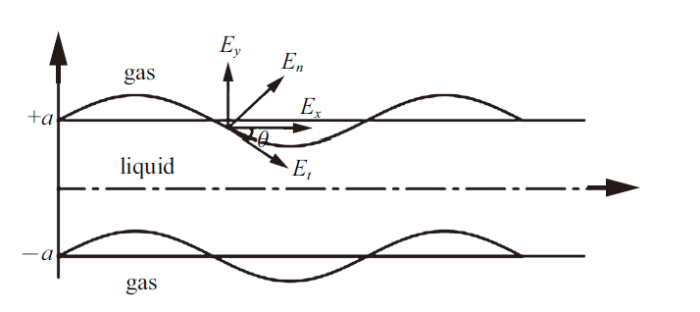Fig.2   Schematic diagram of electric force vector

$\sin\theta =\frac{\partial \eta }{\partial x}\cos\theta =1$

\begin{aligned}T_{\mathrm{t} 1}^{\mathrm{e}}=&\left[\varepsilon_{2} \mathrm{i} k \frac{V_{0}^{2}}{d^{2}} \eta_{0}+\varepsilon_{2} \mathrm{i} k \cdot \frac{V_{0}}{d} P_{3}\left(\mathrm{e}^{k a}+\mathrm{e}^{-k a}\right)\right] \\\mathrm{e}^{\mathrm{i} k x+\omega t}, \quad y=a\end{aligned}
\begin{aligned}T_{\mathrm{t} 2}^{\mathrm{e}}=&\left[-\varepsilon_{2} \mathrm{i} k \frac{V_{0}^{2}}{d^{2}} \eta_{0}+\varepsilon_{2} \mathrm{i} k \cdot \frac{V_{0}}{d} P_{3}\left(\mathrm{e}^{k a}+\mathrm{e}^{-k a}\right)\right] \\\mathrm{e}^{\mathrm{i} k x+\omega t}, \quad y=-a\end{aligned}
\begin{aligned}T_{\mathrm{n} 1}^{\mathrm{e}} &=\left[\varepsilon_{2} \frac{V_{0}^{2}}{d^{3}} \eta_{0}-\varepsilon_{1} k \frac{V_{0}}{d} P_{1}\left(\mathrm{e}^{k a}-\mathrm{e}^{-k a}\right)\right] \\& \mathrm{e}^{\mathrm{i} k x+\omega t}, \quad y=a\end{aligned}

\begin{aligned}u(y) &=B_{1} \mathrm{e}^{l y}+B_{2} \mathrm{e}^{-l y}+\\& \frac{\mathrm{i} k}{\mu\left(k^{2}-l^{2}\right)}\left(A_{1} \mathrm{e}^{k y}+A_{2} \mathrm{e}^{-k y}\right)\end{aligned}
\begin{aligned}v(y) &=-\frac{\mathrm{i} k}{l} B_{1} \mathrm{e}^{l y}+\frac{\mathrm{i} k}{l} B_{2} \mathrm{e}^{-l y}+\\& \frac{k}{\mu\left(k^{2}-l^{2}\right)}\left(A_{1} \mathrm{e}^{k y}-A_{2} \mathrm{e}^{-k y}\right)\end{aligned}

$l^{2}=k^{2}+\frac{\rho}{\mu}(\omega+U \mathrm{i} k)$
\begin{aligned}A_{1}=&-\frac{\mu}{k}\left(k^{2}+l^{2}\right)(\omega+U \mathrm{i} k) \eta_{0} \frac{1}{2 \cosh (k a)}+\\& \varepsilon_{2} k \frac{V_{0}^{2}}{d^{2}} \eta_{0} \frac{1}{2 \sinh (k a)}+\varepsilon_{2} k \frac{V_{0}}{d} P_{3} \operatorname{coth}(k a)\end{aligned}
\begin{aligned}A_{2}=& \frac{\mu}{k}\left(k^{2}+l^{2}\right)(\omega+U \mathrm{i} k) \eta_{0} \frac{1}{2 \cosh (k a)}+\\& \varepsilon_{2} k \frac{V_{0}^{2}}{d^{2}} \eta_{0} \frac{1}{2 \sinh (k a)}+\varepsilon_{2} k \frac{V_{0}}{d} P_{3} \operatorname{coth}(\end{aligned}
\begin{aligned}B_{1}=& \frac{\mathrm{i} k l}{k^{2}-l^{2}}(\omega+U \mathrm{i} k) \eta_{0} \frac{1}{\cosh (l a)}-\\& \frac{\mathrm{i} k l \eta_{0} \varepsilon_{2}}{\mu\left(k^{2}-l^{2}\right)} \frac{V_{0}^{2}}{d^{2}} \eta_{0} \frac{1}{2 \sinh (l a)}-\\& \frac{1}{\mu} \varepsilon_{2} \frac{\mathrm{i} k V_{0}}{d\left(k^{2}-l^{2}\right)} P_{3} \frac{\operatorname{coth}(k a)}{\sinh (l a)}\end{aligned}
\begin{aligned}B_{2}=&-\frac{\mathrm{i} k l}{k^{2}-l^{2}}(\omega+U \mathrm{i} k) \eta_{0} \frac{1}{\cosh (l a)}-\\& \frac{\mathrm{i} k \ln _{0} \varepsilon_{2}}{\mu\left(k^{2}-l^{2}\right)} \frac{V_{0}^{2}}{d^{2}} \eta_{0} \frac{1}{2 \sinh (l a)}-\\& \frac{1}{\mu} \varepsilon_{2} \frac{\mathrm{i} k V_{0}}{d\left(k^{2}-l^{2}\right)} P_{3} \frac{\operatorname{coth}(k a)}{\sinh (l a)}\end{aligned}

$\phi_{\rm g1} =-\frac{1}{k}\omega {\rm e}^{ka-ky}{\rm e}^{{\rm i}kx+\omega t}\eta_{0}$
$p_{\rm g1} =\frac{1}{k}\rho_{\rm g} \omega^{2}{\rm e}^{ka-ky}{\rm e}^{{\rm i}kx+\omega t}\eta_{0}$
$\phi_{\rm g2} =\frac{1}{k}\omega {\rm e}^{-ka+ky}{\rm e}^{{\rm i}kx+\omega t}\eta_{0}$
$p_{\rm g1} =-\frac{1}{k}\rho_{\rm g} \omega^{2}{\rm e}^{-ka+ky}{\rm e}^{{\rm i}kx+\omega t}\eta_{0}$

$\begin{eqnarray} &&{\rm det}(A)=\\&&\quad\left|\begin{array}{c@{\ \ }c@{\ \ }c} \sigma-\varepsilon_{1}(\omega+U{\rm i}k) & \varepsilon_{2}(\omega+U{\rm i}k) & \dfrac{V_{0}}{2 k d^{2}} \sinh (k a) \\ 2 \cosh (k a) & -2 \cosh (k a) & -\dfrac{V_{0}}{d} \\ 0 & D_{32} & D_{33} \end{array}\right|=0\\&& \end{eqnarray}$

\begin{aligned}D_{32} &=-\frac{k^{2}+l^{2}}{k^{2}-l^{2}} \varepsilon_{2} \frac{k V_{0}}{d} 2 \cosh (k a) \operatorname{coth}(k a)+\\& 4 \varepsilon_{2} \frac{k^{2} l V_{0}}{d\left(k^{2}-l^{2}\right)} \frac{\cosh (k a)}{\tanh (l a)}+\varepsilon_{2} \frac{2 k V_{0}}{d} \sinh (k a)\end{aligned}

\begin{aligned}D_{33} &=-\frac{\left(k^{2}+l^{2}\right)^{2}}{k^{2}-l^{2}} \frac{\mu}{k}(\omega+U i k) \tanh (k a)-\\& \frac{k^{2}+l^{2}}{k^{2}-l^{2}} \varepsilon_{2} \frac{k V_{0}^{2}}{d^{2}} \eta_{0} \operatorname{coth}(k a)-\\& 4 \frac{k^{2} l}{k^{2}-l^{2}} \mu(\omega+U i k) \tanh (l a)+\\& 2 \frac{k^{2} l}{k^{2}-l^{2}} \varepsilon_{2} \frac{V_{0}^{2}}{d^{2}} \operatorname{coth}(l a)-\gamma k^{2}-\\& \frac{1}{k} \rho_{\mathrm{g}} \omega^{2}-\varepsilon_{2} \frac{V_{0}^{2}}{d^{3}}\end{aligned}

\begin{aligned}\operatorname{det} A &=\left(\tau-\frac{L^{2}-K^{2}}{R e}\right)(-2 \cosh K) D_{33}+\\& \varepsilon \frac{L^{2}-K^{2}}{R e} \frac{\cosh K}{K D \sinh K} \frac{V_{0}}{d} D_{32}+\\&\left(\tau-\frac{L^{2}-K^{2}}{R e}\right) \frac{V_{0}}{d} D_{32}-\\& \varepsilon \frac{L^{2}-K^{2}}{R e} 2 \cosh K D_{33}=0\end{aligned}

$\begin{array}{c}\frac{V_{0}}{d} D_{32}=-\frac{L^{2}+K^{2}}{K^{2}-L^{2}} 2 \operatorname{coth} K \cosh K \frac{K E u}{D^{2}}+ \\\frac{4 K^{2} L}{K^{2}-L^{2}} \frac{\cosh K}{\tanh L} \frac{E u}{D^{2}}-2 \sinh K \frac{K E u}{D^{2}}\end{array}$

\begin{aligned}D_{33} &=-\frac{\left(L^{2}+K^{2}\right)^{2}}{K} \frac{1}{R e^{2}} \tanh K+\frac{4 K^{2} L}{R e^{2}} \tanh L-\\& \frac{K^{2}}{W e}-\bar{\rho} \frac{\omega^{2}}{K}-\frac{\left(L^{2}+K^{2}\right)^{2}}{K^{2}-L^{2}} \frac{K E u}{D^{2}} \operatorname{coth} K+\\& \frac{2 K^{2} L}{K^{2}-L^{2}} \frac{E u}{D^{2}} \operatorname{coth} L+\frac{E u}{D^{3}}\end{aligned}

\begin{aligned}\operatorname{det} A &=\left(\tau-\frac{L^{2}-K^{2}}{R e}\right)(-2 \sinh K) D_{33}+\\& \varepsilon \frac{L^{2}-K^{2}}{R e} \frac{\sinh K}{K D \cosh K} \frac{V_{0}}{d} D_{32}+\\&\left(\tau-\frac{L^{2}-K^{2}}{R e}\right) \frac{V_{0}}{d} D_{32}-\\& \varepsilon \frac{L^{2}-K^{2}}{R e} 2 \sinh K D_{33}=0\end{aligned}

$\frac{V_{0}}{d} D_{32}=-\frac{L^{2}+K^{2}}{K^{2}-L^{2}} 2 \tanh K \sinh K \frac{K E u}{D^{2}}+\frac{4 K^{2} L}{K^{2}-L^{2}} \frac{\sinh K}{\operatorname{coth} L} \frac{E u}{D^{2}}-2 \cosh K \frac{K E u}{D^{2}}$

\begin{aligned}D_{33} &=-\frac{\left(L^{2}+K^{2}\right)^{2}}{K} \frac{1}{R e^{2}} \operatorname{coth} K+\\& \frac{4 K^{2} L}{R e^{2}} \operatorname{coth} L-\frac{K^{2}}{W e}-\bar{\rho} \frac{\omega^{2}}{K}-\\& \frac{\left(L^{2}+K^{2}\right)^{2}}{K^{2}-L^{2}} \frac{K E u}{D^{2}} \tanh K+\\& \frac{2 K^{2} L}{K^{2}-L^{2}} \frac{E u}{D^{2}} \tanh L+\frac{E u}{D^{3}}\end{aligned}

### 1.3 交流电场作用下不稳定性分析

$\left.\begin{array}{l}\cos \omega t=\frac{1}{2}\left(\mathrm{e}^{\mathrm{i} \Omega t}+\mathrm{e}^{-\mathrm{i} \Omega t}\right) \\\cos ^{2} \omega t=\frac{1}{2}+\frac{1}{4}\left(\mathrm{e}^{2 \mathrm{i} \Omega t}+\mathrm{e}^{-2 \mathrm{i} \Omega t}\right)\end{array}\right\}$

$\begin{array}{c}\{1+\operatorname{Pr}[\cos (\Omega t)-1]\}^{2}=(1-P r)^{2}+\frac{1}{2} P r^{2}+ \\\frac{1}{2} \operatorname{Pr}(1-\operatorname{Pr})\left(\mathrm{e}^{\mathrm{i} \Omega t}+\mathrm{e}^{-\mathrm{i} \Omega t}\right)+\frac{1}{4} \operatorname{Pr}^{2}\left(\mathrm{e}^{2 \mathrm{i} \Omega t}+\mathrm{e}^{-2 \mathrm{i} 2 t}\right)\end{array}$

$D_{n} \hat{\eta}_{n}+x\left(\hat{\eta}_{n+1}+\hat{\eta}_{n-1}\right)+y\left(\hat{\eta}_{n+2}+\hat{\eta}_{n-2}\right)=0$

\begin{aligned}D_{n}=&\left[\left(\tau-\frac{L^{2}-K^{2}}{R e}\right)(-2 \cosh K)-\right.\\&\left.\varepsilon \frac{L^{2}-K^{2}}{R e} 2 \cosh K\right]\left\{\frac{4 K^{2} L}{R e^{2}} \tanh L-\frac{K^{2}}{W e}-\right.\\& \bar{\rho} \frac{w+i n \Omega}{K}+\left(\frac{2 K^{2} L}{K^{2}-L^{2}} \frac{E u}{D^{2}} \operatorname{coth} L+\frac{E u}{D^{3}}-\right.\\&\left.\left.\frac{K^{2}+L^{2}}{K^{2}-L^{2}} \frac{K E u}{D^{2}} \operatorname{coth} K\right) \cdot\left[(1-P r)^{2}+\frac{1}{2} P r^{2}\right]\right\}+\\&\left[\varepsilon \frac{L^{2}-K^{2}}{R e} \frac{\cosh K}{K D \sinh K}+\left(\tau-\frac{L^{2}-K^{2}}{R e}\right)\right] \\&\left(-\frac{K^{2}+L^{2}}{K^{2}-L^{2}} 2 \operatorname{coth} K \cosh K \frac{K E u}{D^{2}}+\frac{4 K^{2} L}{K^{2}-L^{2}}\right.\\&\left.\frac{\cosh K}{\tanh L} \frac{E u}{D^{2}}-2 \sinh K \frac{K E u}{D^{2}}\right) \\&\left[(1-P r)^{2}+\frac{1}{2} P r^{2}\right]\end{aligned}

\begin{aligned}x=\left[\left(\tau-\frac{L^{2}-K^{2}}{R e}\right)(-2 \cosh K)-\right.\\ \left.\varepsilon \frac{L^{2}-K^{2}}{R e} 2 \sinh K\right] \cdot\left(\frac{2 K^{2} L}{K^{2}-L^{2}} \frac{E u}{D^{2}} \operatorname{coth} L+\right.\\ \left.\frac{E u}{D^{3}}-\frac{K^{2}+L^{2}}{K^{2}-L^{2}} \frac{K E u}{D^{2}} \cosh K\right) \cdot \frac{1}{2} \operatorname{Pr}(1-P r)+\\ \left[\varepsilon \frac{L^{2}-K^{2}}{R e} \frac{\cosh K}{K D \sinh K}+\left(\tau-\frac{L^{2}-K^{2}}{R e}\right)\right] \\\left(-\frac{K^{2}+L^{2}}{K^{2}-L^{2}} 2 \operatorname{coth} K \cosh K \frac{K E u}{D^{2}}+\frac{4 K^{2} L}{K^{2}-L^{2}}\right.\\\left.\frac{\cosh K}{\tanh L} \frac{E u}{D^{2}}-2 \sinh K \frac{K E u}{D^{2}}\right) \cdot \frac{1}{2} \operatorname{Pr}(1-P r)\end{aligned}

\begin{aligned}y=&\left[\left(\tau-\frac{L^{2}-K^{2}}{R e}\right)(-2 \cosh K)-\right.\\&\left.\varepsilon \frac{L^{2}-K^{2}}{\operatorname{Re}} 2 \sinh K\right] \cdot\left(\frac{2 K^{2} L}{K^{2}-L^{2}} \frac{E u}{D^{2}} \operatorname{coth} L+\right.\\&\left.\frac{E u}{D^{3}}-\frac{K^{2}+L^{2}}{K^{2}-L^{2}} \frac{K E u}{D^{2}} \cosh K\right) \frac{1}{4} P r^{2}+\\&\left[\varepsilon \frac{L^{2}-K^{2}}{R e} \frac{\cosh K}{K D \sinh K}+\left(\tau-\frac{L^{2}-K^{2}}{R e}\right)\right] \\&\left(-\frac{K^{2}+L^{2}}{K^{2}-L^{2}} 2 \operatorname{coth} K \cosh K \frac{K E u}{D^{2}}+\frac{4 K^{2} L}{K^{2}-L^{2}}\right.\\&\left.\frac{\cosh K}{\tanh L} \frac{E u}{D^{2}}-2 \sinh K \frac{K E u}{D^{2}}\right) \frac{1}{4} P r^{2}\end{aligned}

\begin{aligned}D_{n}=&\left[\left(\tau-\frac{L^{2}-K^{2}}{R e}\right)(-2 \sinh K)-\right.\\&\left.\varepsilon \frac{L^{2}-K^{2}}{R e} 2 \sinh K\right]\left\{\frac{4 K^{2} L}{R e^{2}} \operatorname{coth} L-\frac{K^{2}}{W e}-\right.\\& \bar{\rho} \frac{w+i n \Omega}{K}+\left(\frac{2 K^{2} L}{K^{2}-L^{2}} \frac{E u}{D^{2}} \tanh L+\frac{E u}{D^{3}}-\right.\\&\left.\left.\frac{K^{2}+L^{2}}{K^{2}-L^{2}} \cdot \frac{K E u}{D^{2}} \tanh K\right)\left[(1-P r)^{2}+\frac{1}{2} P r^{2}\right]\right\}+\\&\left[\varepsilon \frac{L^{2}-K^{2}}{R e} \frac{\sinh K}{K D \cosh K}+\left(\tau-\frac{L^{2}-K^{2}}{R e}\right)\right] \\&\left(-\frac{K^{2}+L^{2}}{K^{2}-L^{2}} 2 \tanh K \sinh K \frac{K E u}{D^{2}}+\right.\\&\left.\frac{4 K^{2} L}{K^{2}-L^{2}} \frac{\sinh K}{\operatorname{coth} L} \cdot \frac{E u}{D^{2}}-2 \cosh K \frac{K E u}{D^{2}}\right) \\&\left[(1-P r)^{2}+\frac{1}{2} P r^{2}\right]\end{aligned}

\begin{aligned}x=&\left[\left(\tau-\frac{L^{2}-K^{2}}{R e}\right)(-2 \sinh K)-\right.\\&\left.\varepsilon \frac{L^{2}-K^{2}}{R e} 2 \cosh K\right] \cdot\left(\frac{2 K^{2} L}{K^{2}-L^{2}} \frac{E u}{D^{2}} \tanh L+\right.\\&\left.\frac{E u}{D^{3}}-\frac{K^{2}+L^{2}}{K^{2}-L^{2}} \frac{K E u}{D^{2}} \sinh K\right) \cdot \frac{1}{2} \operatorname{Pr}(1-P r)+\\&\left[\varepsilon \frac{L^{2}-K^{2}}{R e} \frac{\sinh K}{K D \cosh K}+\left(\tau-\frac{L^{2}-K^{2}}{R e}\right)\right] \\&\left(-\frac{K^{2}+L^{2}}{K^{2}-L^{2}} 2 \tanh K \sinh K \frac{K E u}{D^{2}}+\right.\\&\left.\frac{4 K^{2} L}{K^{2}-L^{2}} \frac{\sinh K}{\operatorname{coth} L} \frac{E u}{D^{2}}-2 \cosh K \frac{K E u}{D^{2}}\right) \\& \frac{1}{2} \operatorname{Pr}(1-P r)\end{aligned}

\begin{aligned}y=&\left[\left(\tau-\frac{L^{2}-K^{2}}{R e}\right)(-2 \sinh K)-\right.\\&\left.\varepsilon \frac{L^{2}-K^{2}}{R e} 2 \cosh K\right]\left(\frac{2 K^{2} L}{K^{2}-L^{2}} \frac{E u}{D^{2}} \tanh L+\right.\\&\left.\frac{E u}{D^{3}}-\frac{K^{2}+L^{2}}{K^{2}-L^{2}} \cdot \frac{K E u}{D^{2}} \sinh K\right) \frac{1}{4} P r^{2}+\\&\left[\varepsilon \frac{L^{2}-K^{2}}{R e} \frac{\sinh K}{K D \cosh K}+\left(\tau-\frac{L^{2}-K^{2}}{R e}\right)\right] \\&\left(-\frac{K^{2}+L^{2}}{K^{2}-L^{2}} 2 \tanh K \sinh K \frac{K E u}{D^{2}}+\frac{4 K^{2} L}{K^{2}-L^{2}}\right.\\&\left.\frac{\sinh K}{\operatorname{coth} L} \frac{E u}{D^{2}}-2 \cosh K \frac{K E u}{D^{2}}\right) \frac{1}{4} P r^{2}\end{aligned}

$\left(\begin{array}{c@{\ \ }c@{\ \ }c@{\ \ }c@{\ \ }c@{\ \ }c@{\ \ }c} \ddots & \vdots & \vdots & 0 & 0 & 0 & 0 \\ \ldots & D_{-2} & x_{-1} & y_{0} & 0 & 0 & 0 \\ \ldots & x_{-2} & D_{-1} & x_{0} & y_{1} & 0 & 0 \\ 0 & y_{-2} & x_{-1} & D_{0} & x_{1} & y_{2} & 0 \\ 0 & 0 & y_{-1} & x_{0} & D_{1} & x_{2} & \ldots \\ 0 & 0 & 0 & y_{0} & x_{1} & D_{2} & \ldots \\ 0 & 0 & 0 & 0 & \vdots & \vdots & \ddots \end{array}\right)\left(\begin{array}{c} \hat{\eta}_{-2} \\ \hat{\eta}_{-1} \\ \hat{\eta}_{0} \\ \hat{\eta}_{1} \\ \hat{\eta}_{2} \\ \vdots \end{array}\right)=0$

$\left|\begin{array}{ccccccc}\ddots & \vdots & \vdots & 0 & 0 & 0 & 0 \\\ldots & D_{-2} & x_{-1} & y_{0} & 0 & 0 & 0 \\\ldots & x_{-2} & D_{-1} & x_{0} & y_{1} & 0 & 0 \\0 & y_{-2} & x_{-1} & D_{0} & x_{1} & y_{2} & 0 \\0 & 0 & y_{-1} & x_{0} & D_{1} & x_{2} & \ldots \\0 & 0 & 0 & y_{0} & x_{1} & D_{2} & \ldots \\0 & 0 & 0 & 0 & \vdots & \vdots & \ddots\end{array}\right|=0$

## 2 结果与分析

### 图3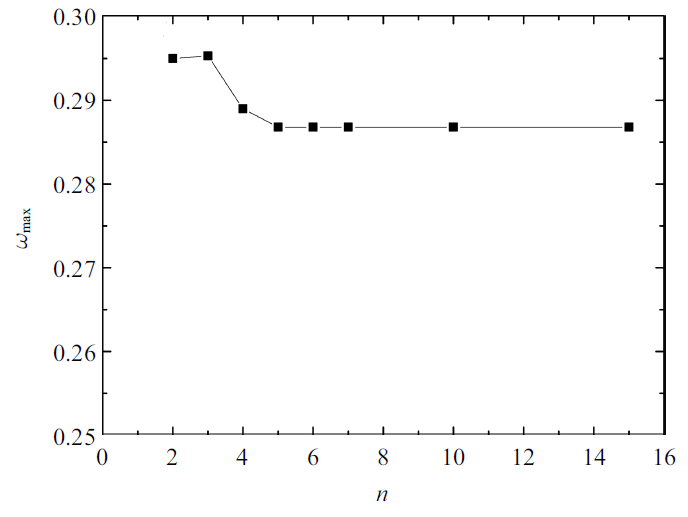Fig.3   $\omega_{\max}$ versus increasing the node number $(\varepsilon =0.012\,5$, $D=10.45$, $Re=1000$, $We=400$, $Eu=5$, $\bar{\rho}=0.001\,2$, $Pr=2/3$, $\varOmega =1$)}

### 图4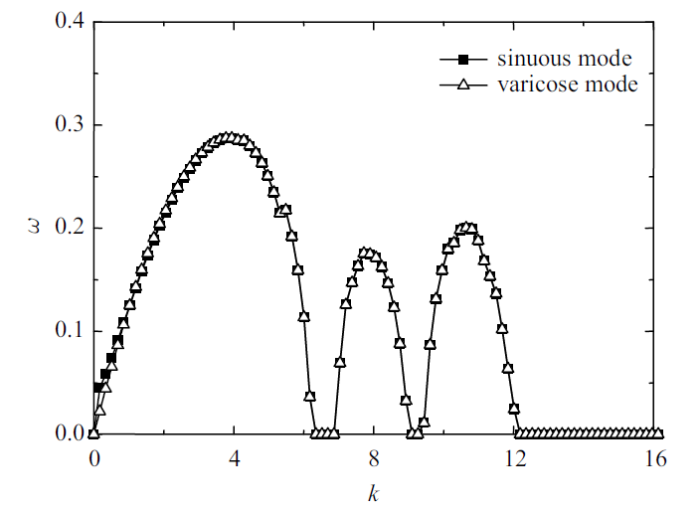Fig.4   Dispersion relation in sinuous and varicose mode ($\varepsilon =0.012 5$, $D=10.45$, $Re=1000$, $We=400$, $Eu=5$, $\bar{\rho}=0.001 2$, $Pr=2/3$, $\varOmega =1$)

### 图5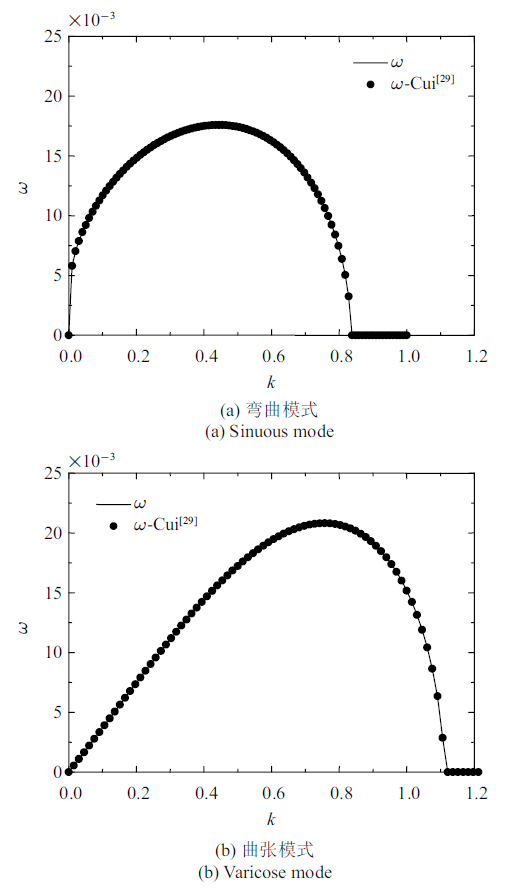Fig.5   Comparison of dispersion relation in DC case $(\varepsilon =0.012\,5$, $D=40$, $Re=1000$, $We=400$, $Eu=2$, $\bar{\rho}=0.001\,2$, $Pr=0$, $\varOmega =1)$

### 图6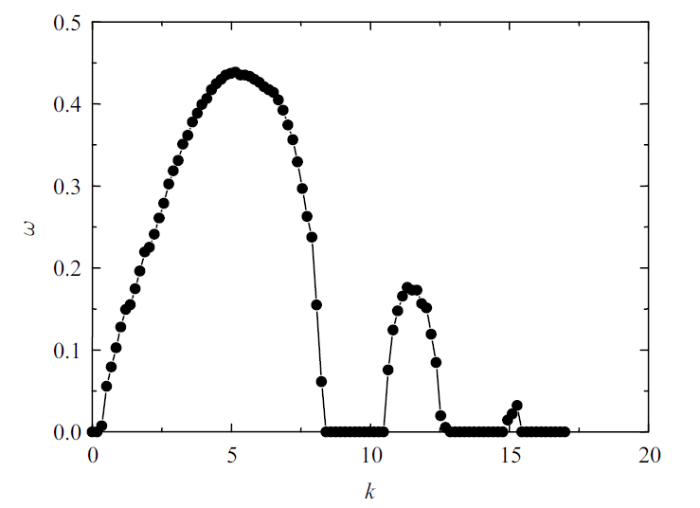Fig.6   Dispersion relation $(\varepsilon =0.012\,5$, $D=40$, $Re=1000$, $We=400$, $Eu=2$, $\bar{\rho}=0.001\,2$, $Pr=0.4$, $\varOmega =1)$

### 图7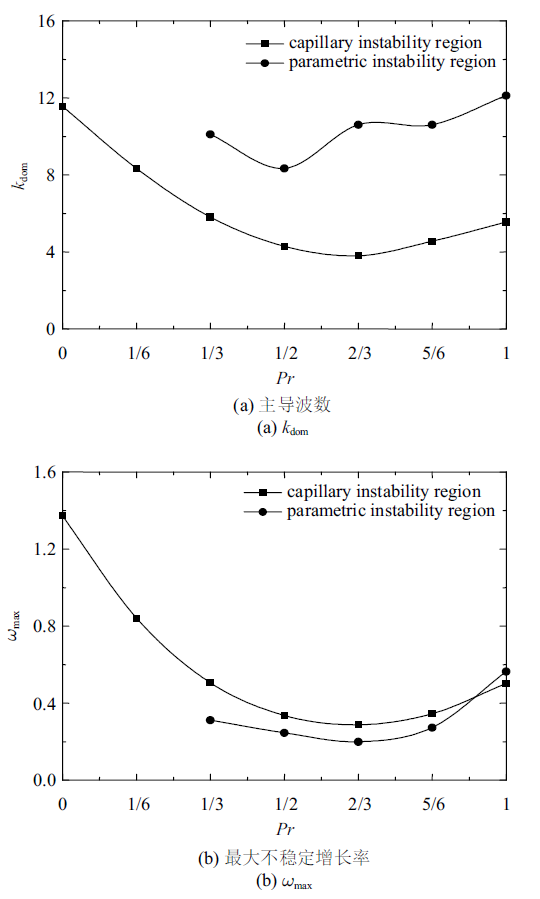Fig.7   Effects of $Pr$ in sinuous mode $(\varepsilon =0.012\,5$, $D=40$, $Re=1000$, $We=400$, $Eu=2$, $\bar{\rho}=0.001\,2$, $\varOmega =1)$

### 图8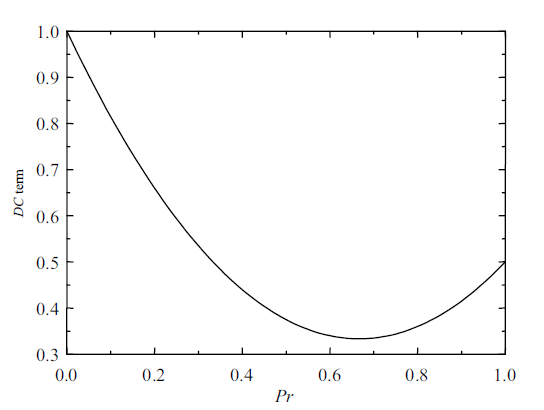Fig.8   Behavior of the DC term $(1-Pr)^{2}+Pr^{2}/2$}

### 图9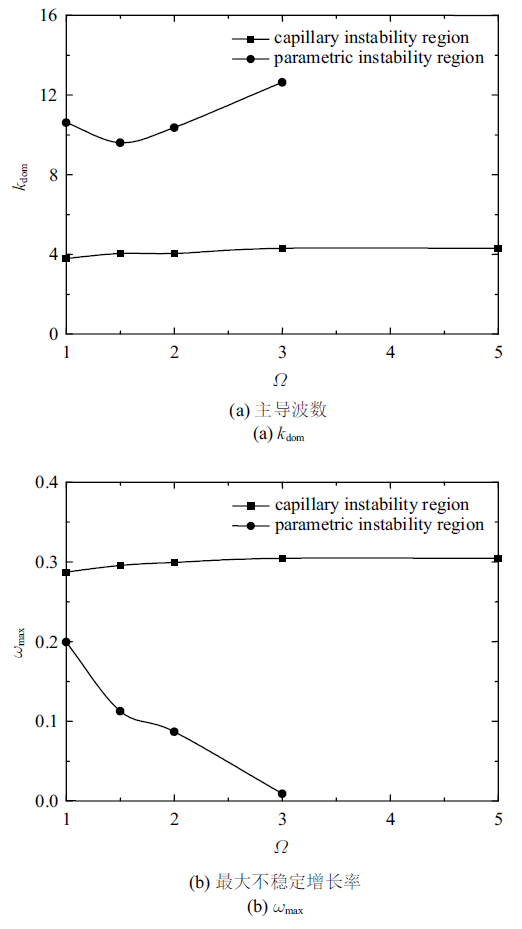Fig.9   Effects of $\varOmega$ in sinuous mode $(\varepsilon =0.012\,5$, $D=40$, $Re=1000$, $We=400$, $Eu=2$, $\bar{\rho}=0.001\,2$, $Pr=2/3)$

### 图10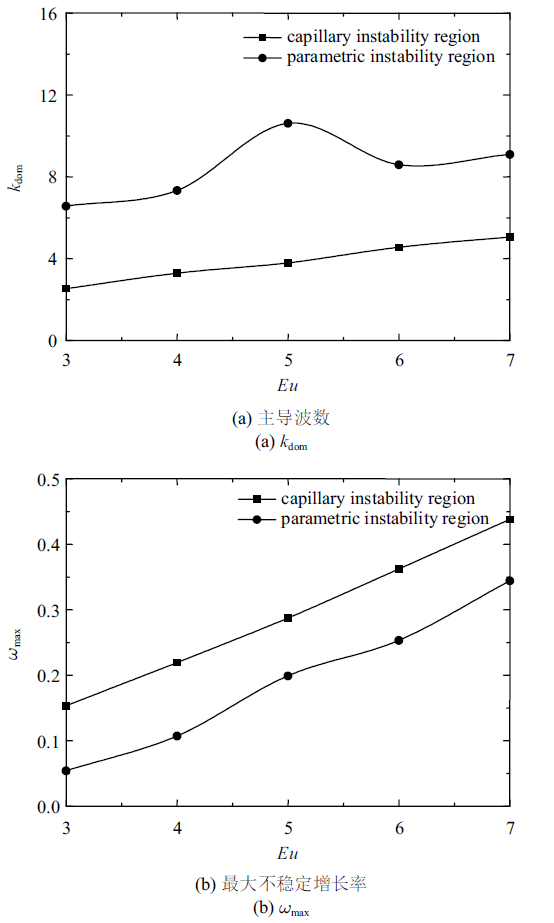Fig.10   Effects of $Eu$ in sinuous mode $(\varepsilon =0.012\,5$, $D=40$, $Re=1000$, $We=400$, $\bar{\rho}=0.001\,2$, $Pr=0.4$, $\varOmega =1)$

## 3 结论

(1) 当交流电场占比$Pr$改变时, 对液膜不稳定性的影响是由于恒定电场比例发生变化所引起的, 此时毛细不稳定性的主导波数和最大不稳定增长率同恒定电场项比例变化趋势相一致, 此时不稳定体现为恒定电场力和表面张力的对抗.

(2) 当交流电场频率$\varOmega$改变时, 交流电场频率的增大急剧地减小液膜的参数不稳定性而略微增大毛细不稳定性.

(3) 当电欧拉数$Eu$发生改变时, 欧拉数的增大同时增大液膜的毛细不稳定性以及参数不稳定性.

(4) 为了在实验中寻求参数振荡现象, 增大电欧拉数$Eu$及减小交流电场频率$\varOmega$是有效的方法.

## 参考文献 原文顺序 文献年度倒序 文中引用次数倒序 被引期刊影响因子

Squire HB.

Investigation of the instability of a moving liquid film

British Journal of Applied Physics, 2002,4(6):167-169

Jones AR, Thong KC.

The production of charged monodisperse fuel droplets by electrical dispersion

Journal of Physics D: Applied Physics, 1971,4(8):1159-1166

Tomita Y, Ishibashi Y, Yokoyama T.

Fundamental studies on an electrostatic ink jet printer: 1st report, electrostatic drop formation

Transactions of the Japan Society of Mechanical Engineers Part B, 1986,29(257):3737-3743

Hagerty WW.

A study of the stability of plane fluid sheets

Journal of Applied Mechanics, 1955,22:509-514

Lin SP.

Stability of a viscous liquid curtain

Journal of Fluid Mechanics, 2006,104:111-118

Li X.

Spatial instability of plane liquid sheets

Chemical Engineering Science, 1993,48(16):2973-2981

Li HS, Kelly RE.

The instability of a liquid jet in a compressible airstream

Physics of Fluids A Fluid Dynamics, 1992,4(10):2162-2168

Cao J, Li X.

Stability of plane liquid sheets in compressible gas streams

Journal of Propulsion & Power, 2011,16(4):623-627

URL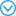( Zhang Peng, Yu Gang.

Rayleigh-Taylor instability of viscous droplets with high Bond numbers

Chinese Journal of Theoretical and Applied Mechanics. 2006,38(3):289-295 (in Chinese))

El-Sayed MF.

Three-dimensional electrohydrodynamic temporal instability of a moving dielectric liquid sheet emanated into a gas medium

The European Physical Journal E, 2004,15(4):443-455

URL( Li Guangbin, Si Ting, Yin Xiezhen.

Temporal instability study of inviscid focused jets under an electric field

Chinese Journal of Theoretical and Applied Mechanics. 2012,44(5):876-883 (in Chinese))

( Li Shuaibing, Yang Rui, Luo Xisheng, et al.

Instability study of an electrified coaxial jet in a coflowing gas stream

Chinese Journal of Theoretical and Applied Mechanics. 2017,49(5):997-1007 (in Chinese))

Yang LJ, Liu YX, Fu QF. et al.

Linear stability analysis of electrified viscoelastic liquid sheets

Atomization & Sprays, 2012,22(11):951-982

The stability of a horizontal fluid interface in a vertical electric field

Journal of Fluid Mechanics, 1965,22(1):1-15

Melcher JR, Warren EP.

Electrohydrodynamics of a current-carrying semi-insulating jet

Journal of Fluid Mechanics, 1971,47(1):127-145

Taylor GI.

Studies in electrohydrodynamics. I. The circulation produced in a drop by electrical field

Proceedings of the Royal Society A, 1966,291(1425):159-166

Fish BR.

Electrical generation of natural aerosols from vegetation

Science, 1972,175(4027):1239-1240

URLIt is suggested that an alternative, or additional, source of the blue haze above heavily forested areas may be the generation of submicrometer-sized wax particles by the action of strong electrical fields at the tips of pine needles and other wax-covered plant surfaces. Exposure of pine needles to high potential gradients results in the production of airborne wax particles with diameters less than 0.6 micrometer.

Saville DA.

Electrohydrodynamics: The Taylor-Melcher leaky dielectric model

Annual Review of Fluid Mechanics, 2003,29(1):27-64

Montanero, José M, Alfonso M, et al.

Dripping, jetting and tip streaming

Reports on Progress in Physics, 2020,83(9):1-60

Gamero-Castaño M, Magnani M.

Numerical simulation of electrospraying in the cone-jet mode

Journal of Fluid Mechanics, 2019,859:247-267

Gamero-Castaño M, Manuel, Magnani M.

The minimum flow rate of electrosprays in the cone-jet mode

Journal of Fluid Mechanics, 2019,876:553-572

Carroll CP, Joo YL.

Electrospinning of viscoelastic boger fluids: Modeling and experiments

Physics of Fluids, 2006,18(5):053102

Reneker DH, Yarin AL.

Electrospinning jets and polymer nanofibers

Polymer, 2008,49(10):2387-2425

URLAbstractIn electrospinning, polymer nanofibers are formed by the creation and elongation of an electrified fluid jet. The path of the jet is from a fluid surface that is often, but not necessarily constrained by an orifice, through a straight segment of a tapering cone, then through a series of successively smaller electrically driven bending coils, with each bending coil having turns of increasing radius, and finally solidifying into a continuous thin fiber. Control of the process produces fibers with nanometer scale diameters, along with various cross-sectional shapes, beads, branches and buckling coils or zigzags. Additions to the fluid being spun, such as chemical reagents, other polymers, dispersed particles, proteins, and viable cells, resulted in the inclusion of the added material along the nanofibers. Post-treatments of nanofibers, by conglutination, by vapor coating, by chemical treatment of the surfaces, and by thermal processing, broaden the usefulness of nanofibers.]]>

Demekhin E, Polyanskikh S, Ramos A.

Taylor cones in a leaky dielectric liquid under an AC electric field

Physical Review E Statal Nonlinear & Soft Matter Physics, 2011,84(3):035301

Yeo LY, Lastochkin D, Wang SC. et al.

A new AC electrospray mechanism by Maxwell-Wagner polarization and capillary resonance

Physical Review Letters, 2004,92(13):133902

Demekhin EA, Polyanskikh SV.

Stability of a viscous liquid jet in a high-frequency alternating electric field

Journal of Applied Mechanics & Technical Physics, 2010,51(1):31-43

Yang W, Duan H, Li C. et al.

Crossover of varicose and whipping instabilities in electrified microjets

Physical Review Letters, 2014,112(5):054501

Ward K, Matsumoto S, Narayanan R.

The electrostatically forced Faraday instability: Theory and experiments

Journal of Fluid Mechanics, 2019,862:696-731

Cui X, Fu QF, Yang LJ. et al.

Linear instability of liquid sheets subjected to a transverse electric field

Journal of Fluids Engineering, 2020,142(1):011203

Jia BQ, Xie L, Yang LJ. et al.

Linear instability of viscoelastic planar liquid sheets in the presence of gas velocity oscillations

Journal of Non-Newtonian Fluid Mechanics, 2019,273:104169

Jia BQ, Xie L, Cui X et al.

Linear stability of confined coaxial jets in the presence of gas velocity oscillations with heat and mass transfer

Physics of Fluids, 2019,31:092101

[博士论文]. 北京: 北京航空航天大学, 2020

( Xie L.

Instability analysis of cylindrical viscous liquid jets under DC and AC electric fields

[PhD Thesis]. Beijing: Beihang University, 2020 (in Chinese))

Benjamin TB, Ursell FJ.

The stability of the plane free surface of a liquid in vertical periodic motion

Proceedings of the Royal Society of London, 1954,225(1163):505-515

( Yao Muwei, Jia Boqi, Yang Lijun, et al.

Instability of power-law liquid sheets in the presence of gas velocity oscillations

Acta Aeronautica et Astronautica Sinica. 2020,41(11):123873 (in Chinese))

( Xie Luo, Yang Lijun.

The instability of liquid jet under radial AC electric field//The 10th Chinese Congress of Theoretical and

Applied Mechanics, 2018,115:92 (in Chinese))

/

 〈〉